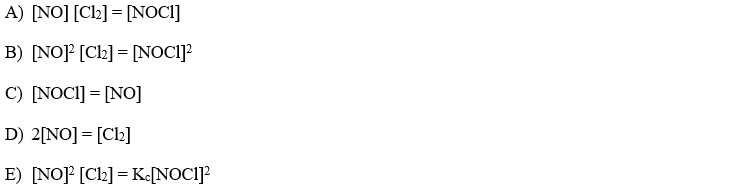# Problem: When the following reaction is at equilibrium, which of these relationships is  always true?2 NOCl (g)  ⇋ 2 NO (g) + Cl 2 (g)

###### Problem Details

When the following reaction is at equilibrium, which of these relationships is  always true?

2 NOCl (g)  ⇋ 2 NO (g) + Cl (g)What scientific concept do you need to know in order to solve this problem?

Our tutors have indicated that to solve this problem you will need to apply the Equilibrium Expressions concept. If you need more Equilibrium Expressions practice, you can also practice Equilibrium Expressions practice problems.

What is the difficulty of this problem?

Our tutors rated the difficulty ofWhen the following reaction is at equilibrium, which of thes...as medium difficulty.

How long does this problem take to solve?

Our expert Chemistry tutor, Dasha took 2 minutes and 22 seconds to solve this problem. You can follow their steps in the video explanation above.

What professor is this problem relevant for?

Based on our data, we think this problem is relevant for Professor Costanza's class at USF.